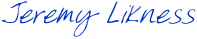## Saturday, October 4, 2014

### AngularJS Tip: Using a Filter with ngClass

I was working on my presentation of building an AngularJS app for the upcoming Atlanta Code Camp and ran into an interesting scenario. The app tracks various variables and formulas and then displays them in a responsive fashion. I’ll post the deck and code after the camp. One formula is Body Mass Index, an indication of general weight and health. I use it as an example but it is not the most accurate and a lean, muscular person may register as overweight or obese on the scale (read my non-technical article 10 Fat Mistakes to learn what you should really focus on).

For the session I want to show a test-driven approach, so I start out by defining what I expect from the formula using Jasmine:

```describe("Formula for BMI", function () {
describe("Given a 5 ft 10 person who weighs 300 pounds", function () {
it("should compute a BMI of 43", function () {            var actual = formulaBmi({                height: 70,                weight: 300            });            expect(actual).toBeCloseTo(43);        });
});
describe("Given a 5 ft 8 in person who weighs 120 pounds", function () {
it("should compute a BMI of 18.2", function () {            var actual = formulaBmi({                height: 68,                weight: 120            });            expect(actual).toBeCloseTo(18.2);        });
});});
```

Next I define the formula and ensure the test passes (notice at this stage I haven’t even involved Angular yet):

```function formulaBmi(profile) {
// BMI = (weight in pound * 703) /     // (height in inches) ^ 2    var bmi = (profile.weight * 703) /        (profile.height * profile.height);
// round it    return Math.round(bmi * 10.0) / 10.0;}```

So that:

• Formula for BMI
• Given a 5 ft 10 person who weighs 300 pounds … should compute a BMI of 43
• Given a 5 ft 8 in person who weighs 120 pounds … should compute a BMI of 18.2

Then I set the expectation it will register with Angular as a service:

```describe("BMI Formula service", function () {    var formulaBmiSvc;
beforeEach(function () {        module('healthApp');    });
beforeEach(inject(function (formulaBmiService) {        formulaBmiSvc = formulaBmiService;    }));
it("should be defined", function () {        expect(formulaBmiSvc).not.toBeNull();    });
it("should be a function", function () {        var fnPrototype = {},            isFn = formulaBmiSvc &&            fnPrototype.toString.call(formulaBmiSvc)            === '[object Function]';        expect(isFn).toBe(true);    })});
```

… and I register the function with Angular to make the test pass:

```(function (app) {
app.factory('formulaBmiService', function () {        return formulaBmi;    });
})(angular.module('healthApp'));```

So that:

• BMI Formula Service
• should be defined
• should be a function

To show the index I want to translate it to the scale it provides. Anything under than 18.5 is considered underweight, while anything over 25 is overweight and over 30 is considered obese.

Now I can write the tests for a filter that satisfies these conditions:

```describe("BMI filter", function () {    var bmiFilter;
beforeEach(function () {        module('healthApp');    });
beforeEach(inject(function (\$filter) {        bmiFilter = \$filter('bmi');    }));
it("should be defined", function () {        expect(bmiFilter).not.toBeNull();    });
describe("Given BMI is less than 18.5", function () {        it("should return Underweight", function () {            var actual;            actual = bmiFilter(18.4);            expect(actual).toBe('Underweight');        });    });
describe("Given BMI is greater than or equal to 18.5 and less than 25", function () {        it("should return Normal", function () {            var actual;            actual = bmiFilter(18.6);            expect(actual).toBe('Normal');        });    });
describe("Given BMI is greater than or equal to 25 and less than 30", function () {        it("should return Overweight", function () {            var actual;            actual = bmiFilter(26);            expect(actual).toBe('Overweight');        });    });
describe("Given BMI is greater than or equal to 30", function () {        it("should return Obese", function () {            var actual;            actual = bmiFilter(31);            expect(actual).toBe('Obese');        });    });
});
```

… and the filter itself:

```(function (app) {
app.filter('bmi', function () {        return function (input) {            var value = Number(input);
if (value >= 30.0) {                return 'Obese';            }
if (value >= 25.0) {                return 'Overweight';            }
if (value < 18.5) {                return 'Underweight';            }
return 'Normal';        };    });
})(angular.module('healthApp'));```

So that:

• BMI filter
• should be defined
• Given BMI is less than 18.5 … should return Underweight
• Given BMI is greater than or equal to 18.5 and less than 25 … should return Normal
• Given BMI is greater than or equal to 25 and less than 30 … should return Overweight
• Given BMI is greater than or equal to 30 … should return Obese

Now that I have the filter, I can use it to show the text for the current BMI:

`<h1>BMI:</h1><h2>{{ctrl.bmiValue}}</h2><b>{{ctrl.bmiValue | bmi}}</b>`

This will show the title, the actual numeric BMI value and the designation of Underweight, Normal, Overweight, or Obese.

The problem I have is that I want to apply a class and show it in light red for the underweight and overweight conditions, and dark red for the obese condition. I could use ng-class and simply redefine the ranges like this:

```<div ng-class="{ obese: ctrl.bmiValue >= 30 }"></div>
```

… but that feels like duplicating code. Fortunately, the ng-class directive supports having an expression passed in. What it evaluates to is what it will use as the class. So, I simply define it like this:

`<div class="tile"     ng-class="ctrl.bmiValue | bmi"     title="Body Mass Index">...</div>`

And ensure my CSS is set up correctly :

```div.Obese {    background: red;}

div.Overweight {    background: lightcoral;}

div.Underweight {    background: lightcoral;}
```

Now I’m good to go. If you are concerned that the classes don’t follow convention (they are uppercase) and maybe need a more unique name (such as a prefix to avoid collisions) the solution is simple: add a parameter to the filter and based on that parameter, return the readable text or the translated class. Either way you encapsulate the logic in one place for reuse throughout your app.

Here’s a snap of the result scaled to a mobile form factor:

If you are in the Atlanta area and reading this before October 11, 2014, please come join us at the code camp. It is a day loaded with great sessions. Until then, enjoy your Angular coding!